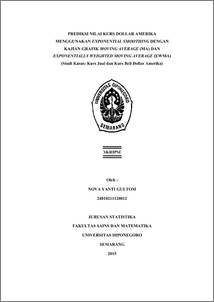# PREDIKSI NILAI KURS DOLLAR AMERIKA MENGGUNAKAN EXPONENTIAL SMOOTHING DENGAN KAJIAN GRAFIK MOVING AVERAGE (MA) DAN EXPONENTIALLY WEIGHTED MOVING AVERAGE (EWMA) (Studi Kasus: Kurs Jual dan Kurs Beli Dollar Amerika)

GULTOM, NOVA YANTI (2015) PREDIKSI NILAI KURS DOLLAR AMERIKA MENGGUNAKAN EXPONENTIAL SMOOTHING DENGAN KAJIAN GRAFIK MOVING AVERAGE (MA) DAN EXPONENTIALLY WEIGHTED MOVING AVERAGE (EWMA) (Studi Kasus: Kurs Jual dan Kurs Beli Dollar Amerika). Undergraduate thesis, FSM Universitas Diponegoro.Preview
PDF
6Mb

## Abstract

The exchange rate is an exchange between two different currencies, it will receive the value or price comparisons between two currencies. It is to determine the predictive value of the exchange rate in the next period is done by using Exponential Smoothing. The quality control can be done by forming graphics controllers. The exchange rate can be done in small shifts, so the exchange rate can use graphics controller Moving Average and Exponentially Weighted Moving Average (EWMA). At the selling rate is found value trial and error alpha is 0,9 and gamma is 0,01 with value of MAPE is 0,37; MAD is 46,94 and value of MSD is 4515,27. At the buying rate is found value trial and error alpha is 0,84 and gamma is 0,01 with value of MAPE is 0,37; MAD is 46,57 and value of MSD is 4524,48. In the graph MA and EWMA most sensitive is the MA control chart so in the weekly chart MA selling rate with w is 5 and L is 2,8 obtainable UCL is 13132,52; CL is 12654, LCL is 12175,47. On the weekly chart MA buying rate with w is 5 and L is 2,8 obtainable UCL is 13002,08; CL is 12528, LCL is 12053,91. Then the possibility of the exchange rate for the next period will be increased or decreased to the rupiah. Keywords: Exchange Rate, Exponential Smoothing, Graphic control, Moving Average (MA), Exponentially Weighted Moving Average (EWMA).

Item Type: Thesis (Undergraduate) H Social Sciences > HA Statistics Faculty of Science and Mathematics > Department of Statistics 47457 Mr Hasbi Yasin 01 Feb 2016 12:08 01 Feb 2016 12:08

Repository Staff Only: item control page